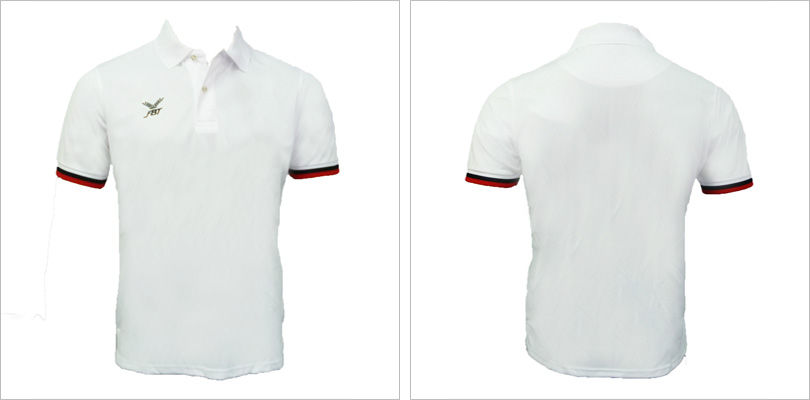## A Kind Gesture

A Start To Their Dream

Singapore Disability Sports Council (SDSC) athletes need the items listed to enhance their trainings. You may do a part by adopting some of the items and bring it down to Siglap South CC between 1 June- 29 June.

## scroll### Size: S (x5) M (x4) L (x4) XL (x2)### Size: S (x5) M (x4) L (x4) XL (x2)### Size: S (x5) M (x4) L (x4) XL (x2)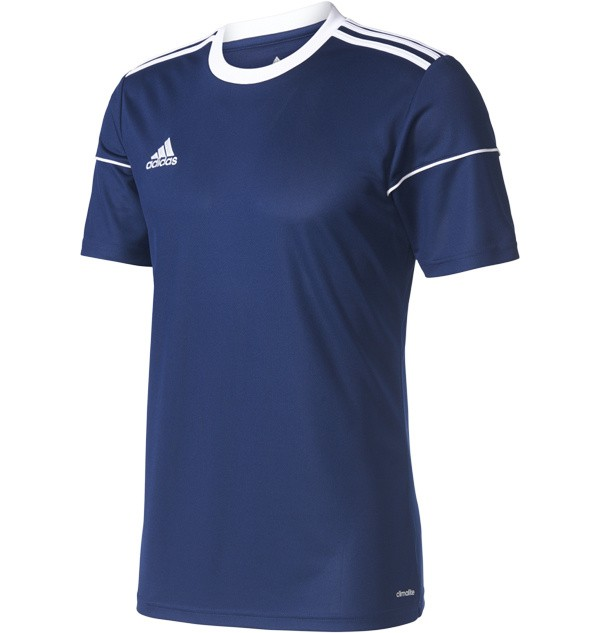### Size: S (x5) M (x4) L (x4) XL (x2)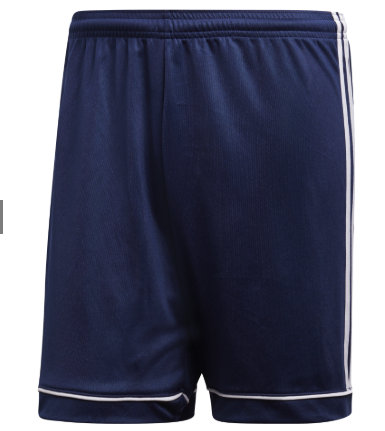### Size: S (x5) M (x4) L (x4) XL (x2)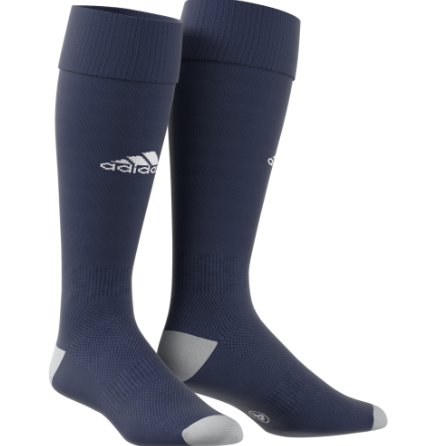### Size: S (x5) M (x4) L (x4) XL (x2)### Size: S (x5) M (x4) L (x4) XL (x2)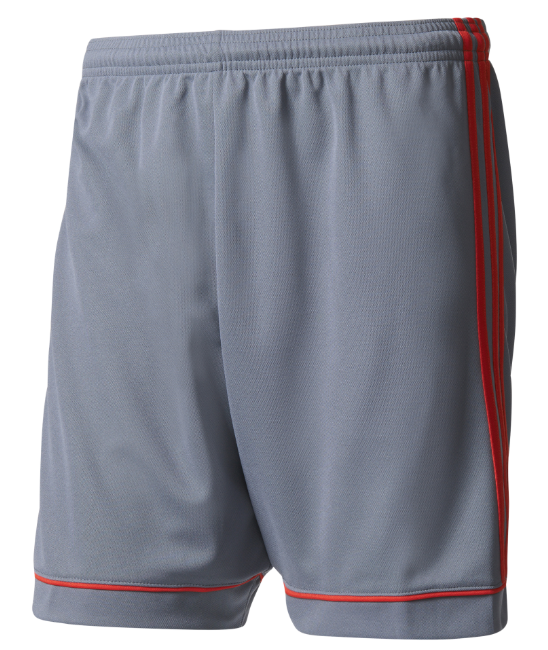### Size: S (x5) M (x4) L (x4) XL (x2)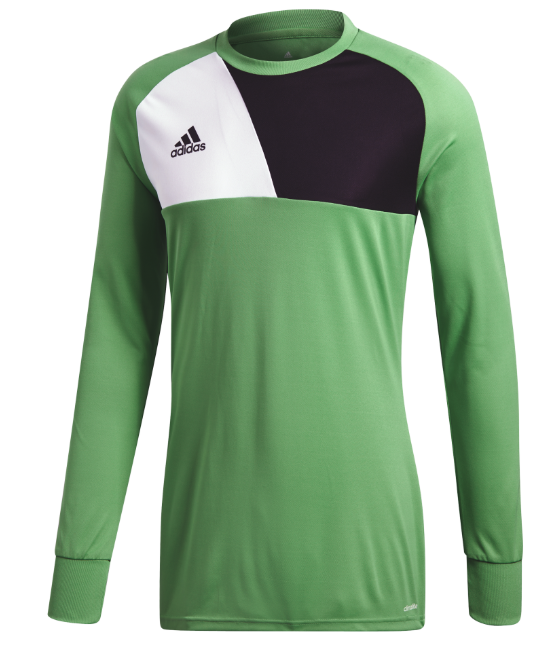### Size: S (x2) M (x2) L (x2) XL (x2)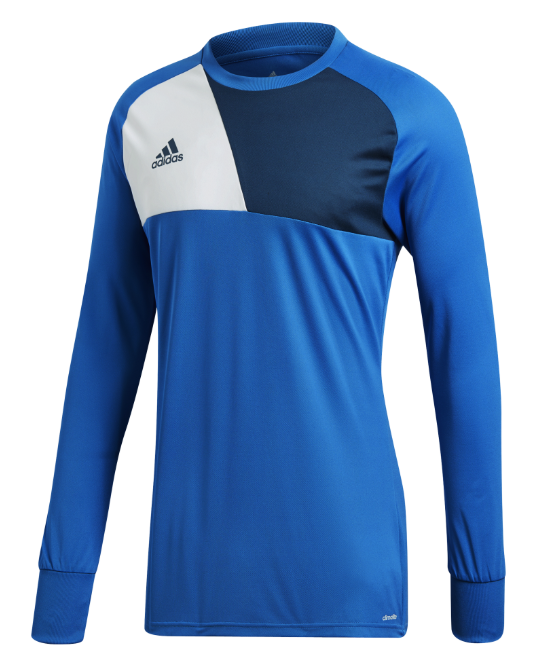### Size: S (x2) M (x2) L (x2) XL (x2)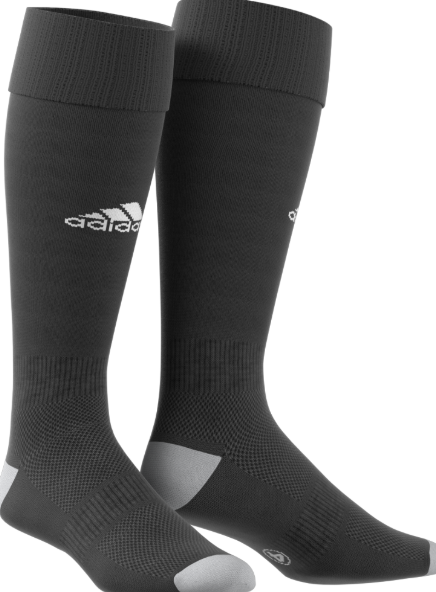### Size: S (x2) M (x2) L (x2) XL (x2)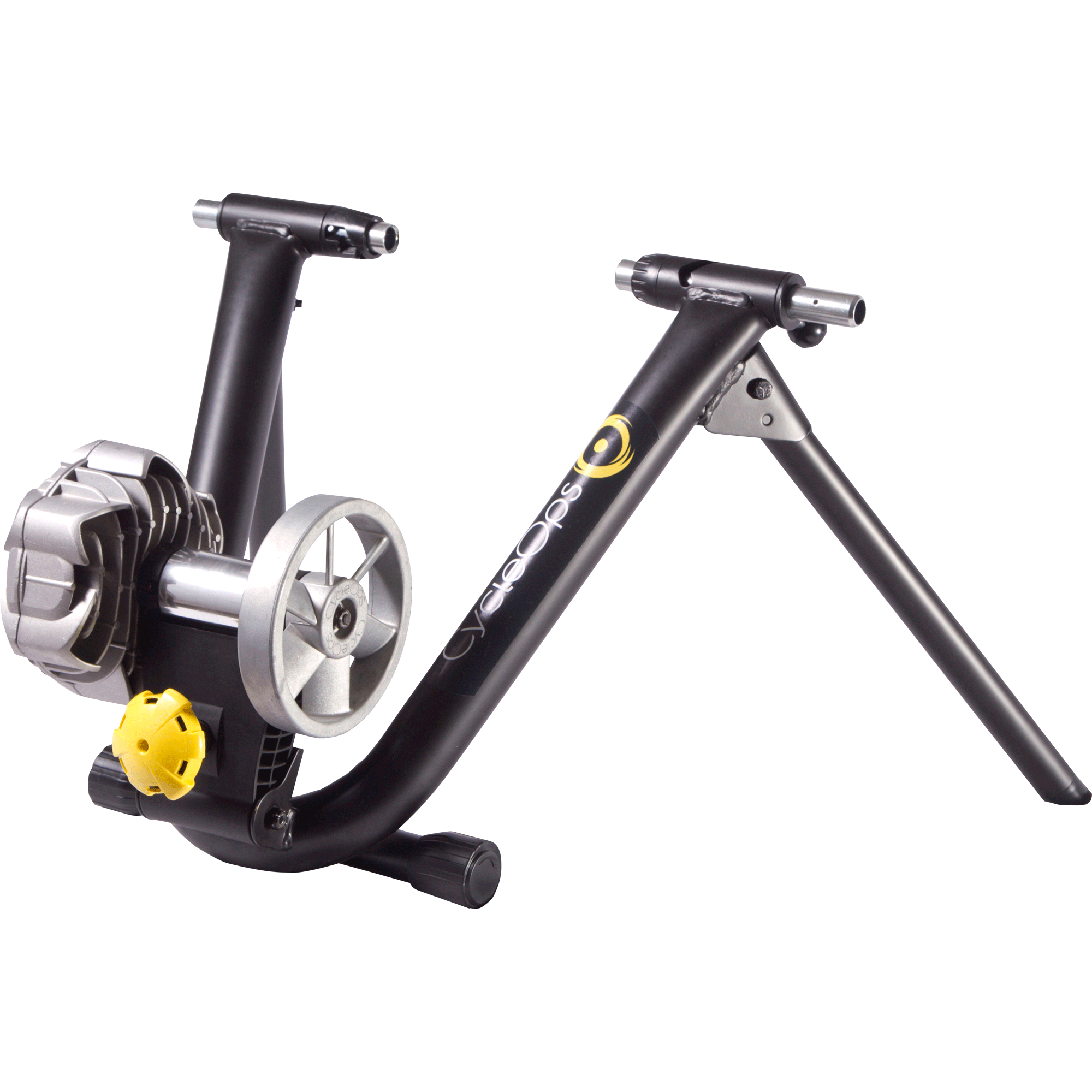### 4 Bike Trainers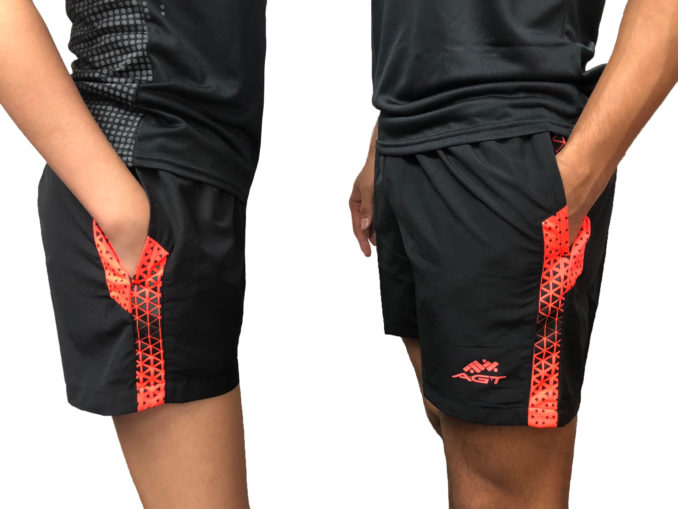### Sizes: XS (x5) S (x15) M (x15) L (x15) XL (x10) XXL (x10) XXXL (x4)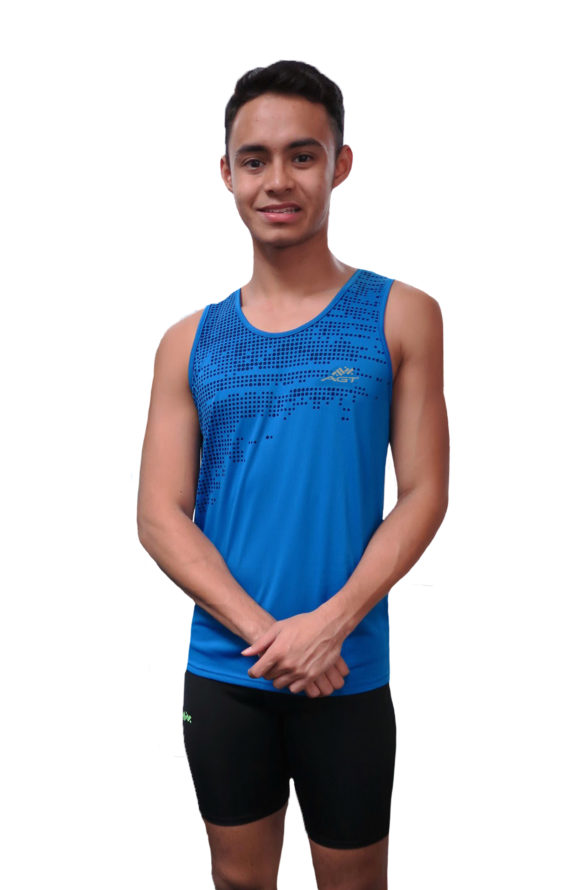### Sizes: XS (x5) S (x15) M (x15) L (x15) XL (x10) XXL (x10) XXXL (x4)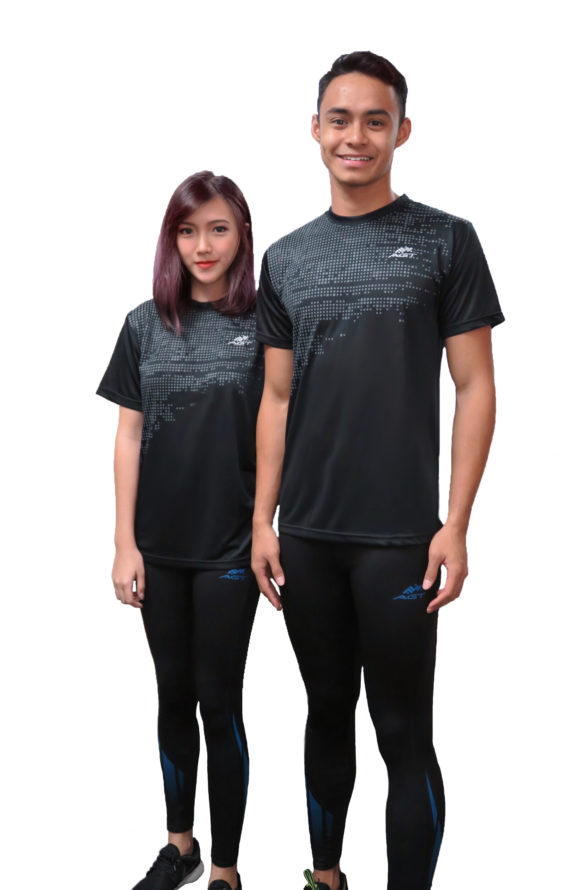### Sizes: XS (x5) S (x15) M (x15) L (x15) XL (x10) XXL (x10) XXXL (x4)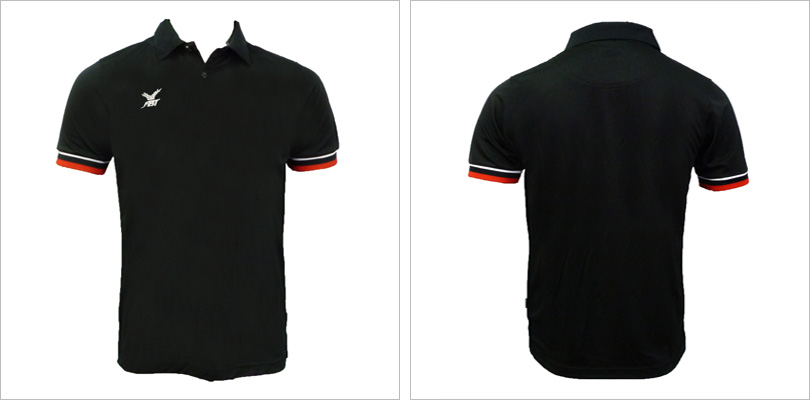### Sizes: S (x5) M (x10) L (x10) XL (x5)